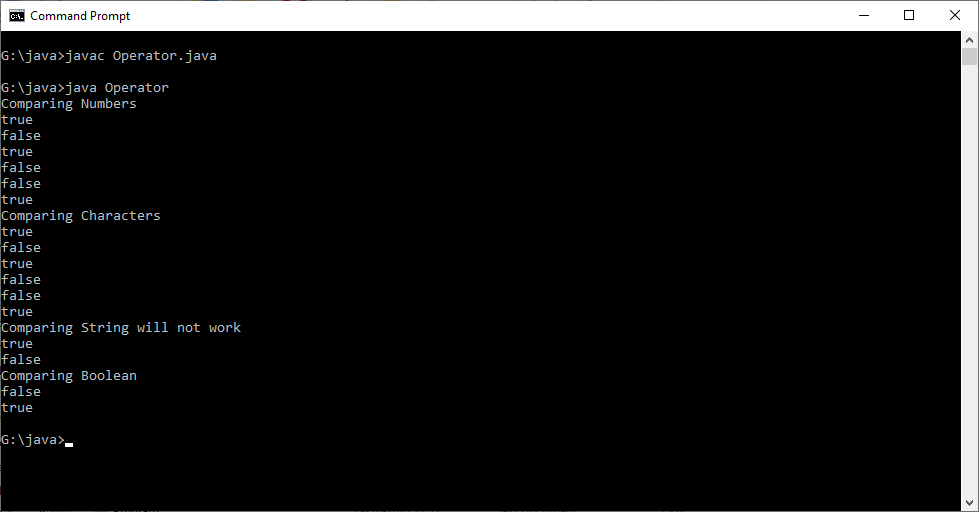# Relational Operators Example in java

Write a Java  Program which illustrates the relational operators, it purpose, and supported data types.

Strongly recommended to Solve it on your own, Don't directly go to the solution given below.

public class Hello { public static void main(String args[]) { //Write your code here } }

#### Program or Solution

``` import java.util.Scanner;class Operator{ public static void main(String args[]) { int num_1 = 10; int num_2 = 20; char ch_1 = 'D'; char ch_2 = 'E'; String name_1 = "Decode"; String name_2 = "Decode"; boolean val_1 = true; boolean val_2 = false; //Scanner sc = new Scanner(System.in); //name_2 = sc.next(); //Comparing Numbers like int and float are acceptable System.out.println("Comparing Numbers"); System.out.println(num_1<num_2); System.out.println(num_1>num_2); System.out.println(num_1<=num_2); System.out.println(num_1>=num_2); System.out.println(num_1==num_2); System.out.println(num_1!=num_2); //Comparing Characters are acceptable System.out.println("Comparing Characters"); System.out.println(ch_1<ch_2); System.out.println(ch_1>ch_2); System.out.println(ch_1<=ch_2); System.out.println(ch_1>=ch_2); System.out.println(ch_1==ch_2); System.out.println(ch_1!=ch_2); //Comparing Strings are not possible with Strings. System.out.println("Comparing String will not work"); //System.out.println(name_1<name_2); //System.out.println(name_1>name_2); //System.out.println(name_1<=name_2); //System.out.println(name_1>=name_2); System.out.println(name_1==name_2); // However this is not right method to compare Strings. System.out.println(name_1!=name_2); // However this is not right method to compare Strings.                         //Comparing Booleans "equal to" and "not equal to" are allowed System.out.println("Comparing Boolean"); //System.out.println(val_1<val_2); //System.out.println(val_1>val_2); //System.out.println(val_1<=val_2); //System.out.println(val_1>=val_2); System.out.println(val_1==val_2); System.out.println(val_1!=val_2); }} ```

#### Output#### Program Explanation

Relational operators or conditional operators are used to compare two operands and returns binary value.

Comparisons include equality, inequality, greater, and lesser. Relational operators return true if the comparison is correct, else return false.

Relational Operators can be used with following data types.

 Operator Integer/Long Float/Double Character String Boolean Equal to (==) ✓ ✓ ✓ ✓(Does not compareStrings. It compare String Location address) ✓ Not Equal to (!=) ✓ ✓ ✓ ✓(Does not compareStrings. It compare String Location address) ✓ Less than (<) ✓ ✓ ✓ Greater than (>) ✓ ✓ ✓ Less than or Equal to (<=) ✓ ✓ ✓ Greater than or Equal to (>=) ✓ ✓ ✓

== does not compare two strings, instead compare memory location of two strings. To understand remove the // in the following lines and give "Decode" as input and check.

`//Scanner sc = new Scanner(System.in);//name_2 = sc.next();`

it returns false even though both of the string variables has "Decode".